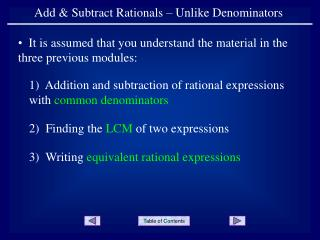DownloadDownload PresentationAdd & Subtract Rationals – Unlike Denominators

# Add & Subtract Rationals – Unlike Denominators

Download Presentation## Add & Subtract Rationals – Unlike Denominators

- - - - - - - - - - - - - - - - - - - - - - - - - - - E N D - - - - - - - - - - - - - - - - - - - - - - - - - - -
##### Presentation Transcript

1. Add & Subtract Rationals – Unlike Denominators • It is assumed that you understand the material in the three previous modules: 1) Addition and subtraction of rational expressions with common denominators 2) Finding the LCM of two expressions 3) Writing equivalent rational expressions

2. To add or subtract rational expressions with unlikedenominators: 1) Determine the LCD (LCM of the denominators) 2) Write each rational expression as an equivalent rational expression with the LCD as its denominator 3) Add or subtract as you did with common denominators

3. Example 1 Add the two rational expressions Note that the denominators are not common Determine the LCD

4. Write each rational as an equivalent expression with the LCD

5. Write each rational as an equivalent expression with the LCD

6. Write each rational as an equivalent expression with the LCD for the denominator

7. Add the equivalent rational expressions that now have a common denominator

8. Since we can’t factor the numerator and nothing can reduced, this is the answer.

9. Example 2 In the last problem, we went to great detail to find the equivalent rational expressions. Here is a shorter version of the same problem. Add the two rational expressions Determine the LCD

10. Write the equivalent rational expressions

11. Since we can’t factor the numerator and nothing can be reduced, this is the answer.

12. Example 3 Subtract the two rational expressions Note that the denominators are not common Determine the LCD

13. Write the equivalent rational expressions

14. Since we can’t factor the numerator and nothing can be reduced, this is the answer.

15. Example 4 Subtract the two rational expressions Note that the denominators are not common Determine the LCD

16. Be careful of the sign here

17. END OF PRESENTATION Click to rerun the slideshow.